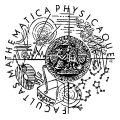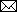Document sans titreResearch Team1 MUUK Charles University Institute of Mathematics KNM Department of Computational Mathematics KMA Department of Mathematical Analysis Sokolovská 83, 186 75 Praha 8

Permanent researchers
_______________________________________________________

BÁRTA Tomáš
• RESEARCH INTERESTS
• Integro-differential partial differential equations
DOLEJŠÍ Vit
• RESEARCH INTERESTS
• Finite element and finite volume methods, scientific computing of compressible fluid flow
FEISTAUER Miloslav
• RESEARCH INTERESTS
• Numerical methods for PDE's, finite element methods, discontinuous Galerkin method, finite volume method, numerical methods for the simulation of compressible flows
FELCMAN Jiří
• RESEARCH INTERESTS
• Numerical methods for the simulation of compressible flows
HASLINGER Jaroslav
• RESEARCH INTERESTS
• Shape optimization, contact problems, finite element methods, variational inequalities
HRON Jaroslav
• RESEARCH INTERESTS
• partial differential equations, equations of continuum physics
• RESEARCH INTERESTS
• Bifurcation theory, numerical methods, partial differential equations, viscoelasticity
KAPLICKÝ Petr
• RESEARCH INTERESTS
• Regularity of nonlinear partial differenatial equations
KNOBLOCH Petr
• RESEARCH INTERESTS
• Finite element method, stabilization techniques, convection-diffusion equations, incompressible flow problems, fluid-structure interaction
MÁLEK Josef
• RESEARCH INTERESTS
• partial differential equations, equations of continuum physics
MALÝ Jan
• RESEARCH INTERESTS
• Real analysis, calculus of variations, functional analysis, partial differential equations, measure theory
PICK Luboš
• RESEARCH INTERESTS
• Function spaces, interpolation theory
POKORNÝ Milan
• RESEARCH INTERESTS
• Navier-Stokes equations, partial differential equations
PRAŽÁK Dalibor
• RESEARCH INTERESTS
• Dynamical systems, long-time behavior, differential equations
ROUBÍČEK Tomáš
• RESEARCH INTERESTS
• Nonlinear partial differential equations, optimal control, models of continuum mechanics, calculus of variations

Visiting researchers
________________________________________________

_____Senior researchers
_____Junior researchers
BULICEK Miroslav
•• Visiting Center from 01/10/06 to 31/12/07
• RESEARCH INTERESTS
• Partial differential equations; incompressible fluids with temperature, pressure and shear-rate dependent viscosity; long-time behavior of flows of incompressible non-Newtonian fluids; relation between weak and Lagrangian formulation
PANAGIOTOPOULOS Christos
•• Visiting Center from 06/10/10 to 06/11/10
• RESEARCH INTERESTS
• Computational Methods in Engineering, Fracture mechanics, Wave Propagation and Elastodynamics, Energetic formulations for Rate-Independent Processes in Continuum Mechanics, Software Development for Computational Mechanics
ROSSI Riccarda
•• Visiting Center from 15/03/09 to 15/04/09
• Previous stays:
from 01/12/08 to 31/12/08
• RESEARCH INTERESTS
• -analysis of abstract rate-independent systems -analysis of abstract evolution equations in metric and Banach spaces -rate-independent and rate-dependent models of adhesive contact -analysis of phase transition problems
SOUCEK Ondrej
•• Visiting Center from 01/01/11 to 31/12/11
• RESEARCH INTERESTS
• Application of continuum thermodynamics of mixtures to ice-sheet dynamics, approximative techniques for ice flow problem Earths electromagnetic field: Inverse modeling and adjoint-problem for 3-D electric conductivity of the Earths mantle
WACHSMUTH Daniel
•• Visiting Center from 12/03/07 to 12/04/07
• RESEARCH INTERESTS
• I would like to work on optimal control of Navier-Stokes equations with non-standard boundary conditions. Optimal control problems for non-Newtonian fluids would also be a challenging topic. I follow an invitation of Prof. Roubicek.
WELLER Frederic
•• Visiting Center from 22/10/07 to 22/12/07
• RESEARCH INTERESTS
• Influence of shear stress on primary hemostasis. Classical solvability of a free boundary problem modeling thrombus growth. Modeling of biol. & techn. processes. Fluid Dynamics, elliptic & parabolic PDEs, nonl. functional analysis.
_____Ph.D. students
BENESOVA Barbora
•• Visiting Center from 01/10/08 to 31/03/09
• RESEARCH INTERESTS
• I am interested in mathematical modeling of shape-memory materials which is connected to the issue of relaxation of functionals mainly by means of Young measures. Besides I am concerned with numerical implementations of this model.
FINDEJS Karel
•• Visiting Center from 01/02/08 to 31/07/08
• Previous stays:
from 01/10/07 to 31/12/07
• RESEARCH INTERESTS
• Higher-order methods for solving equations of fluid dynamics Finite volume methods with Higher-order polynomial reconstruction ADER approach for increasing the order in time Combination of higher-order methods with mesh adaptivity
HNETYNKOVA Iveta
•• Visiting Center from 01/07/06 to 31/12/06
• RESEARCH INTERESTS
• The main area of my research is solving systems of linear algebraic equations Ax=b with a large, sparse, nonsingular matrix. I concentrate on accelerating convergence of iterative methods (Krylov subspace methods) by using preconditioning.
•